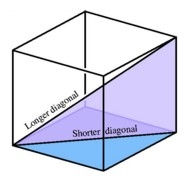# Space diagonal

The space diagonal of a cube is 129.91 mm. Find the lateral area, surface area and the volume of the cube.

L =  5625 mm2
S =  33750 mm2
V =  421875 mm3

### Step-by-step explanation:Did you find an error or inaccuracy? Feel free to write us. Thank you!Tips to related online calculators
Do you want to convert length units?
Tip: Our volume units converter will help you with the conversion of volume units.
Pythagorean theorem is the base for the right triangle calculator.

#### You need to know the following knowledge to solve this word math problem:

We encourage you to watch this tutorial video on this math problem:

## Related math problems and questions:

• Cube diagonalDetermine the length of the cube diagonal with edge 37 mm.
• Cube wallThe perimeter of one cube wall is 120 meters. Calculate the surface area and the body diagonal of this cube.
• Cube diagonalsDetermine the volume and surface area of the cube if you know the length of the body diagonal u = 216 cm.
• Cone - sideFind the cone's surface area and volume if its height is 125 mm and the side length is 17 cm.
• Body diagonal - cubeCalculate the surface and cube volume with body diagonal 15 cm long.
• Surface and volume - cubeFind the surface and volume of a cube whose wall diagonal is 5 cm long.
• Body diagonalFind the cube surface if its body diagonal has a size of 6 cm.
• Center of the cubeThe Center of the cube has a distance 16 cm from each vertex. Calculate the volume V and surface area S of the cube.
• Cube wallCalculate the cube's diagonal if you know that one wall's surface is equal to 36 centimeters square. Please also calculate its volume.
• CubeCalculate the surface of the cube ABCDA'B'C'D' if the area of rectangle ACC'A' = 344 mm2.
• Body diagonalCalculate the volume and surface of the cube if the body diagonal measures 10 dm.
• Regular square prismThe volume of a regular square prism is 192 cm3. The size of its base edge and the body height is 1: 3. Calculate the surface of the prism.
• Lateral surface areaThe ratio of the area of the base of the rotary cone to its lateral surface area is 3: 5. Calculate the surface and volume of the cone, if its height v = 4 cm.
• Sphere areaA cube with an edge 1 m long is a circumscribed sphere (vertices of the cube lie on a sphere's surface). Find the surface area of the sphere.
• Body diagonalCalculate the cube volume, whose body diagonal size is 75 dm. Draw a picture and highlight the body diagonal.
• Rotating coneFind the rotating cone's surface and volume if its side is 150 mm long and the circumference of the base is 43.96 cm.
• Cube and sphereCube with the surface area 150 cm2 is described sphere. What is sphere surface?